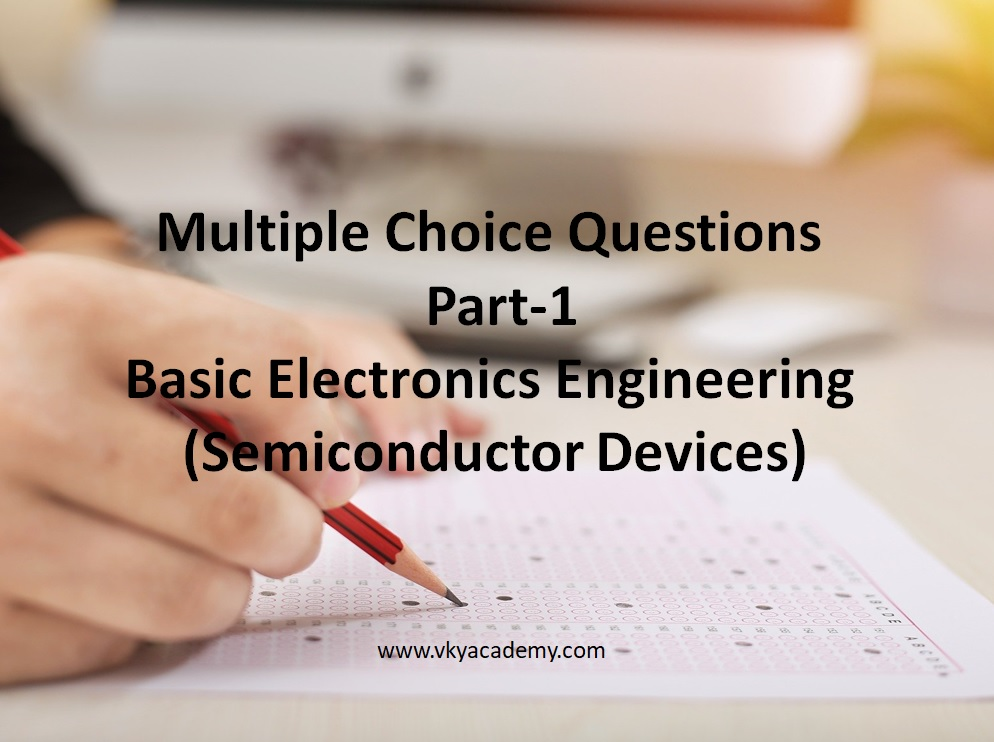# Basic Electronic Engineering Quiz – Part-1 (Semiconductor Devices and PN Junction diode)

Welcome to your %Basic Electronics Quiz%

1. During reverse bias, a small current develops known as:
2. How does a semiconductor behave at absolute zero?
3. What are the charge carriers in semiconductors?
4. How are charge carriers produced in intrinsic semiconductors?
5. What type of material is obtained when an intrinsic semiconductor is doped with pentavalent impurity?
6. What type of material is obtained when an intrinsic semiconductor is doped with trivalent impurity?
7.In a PN junction the potential barrier is due to the charges on either side of the junction, these charges are
8. For a PN junction diode, the current in reverse bias maybe
9.When PN junction is in forward bias, by increasing the battery voltage
10. When a PN junction is reverse biased
11. A PN junction
12. A crystal diode has ………
13. The conductivity of a semiconductor increases with
14. The threshold voltage Vt of Silicon type PN junction diode is
15. The Knee voltage Vk of Silicon type PN junction diode is
16. The threshold voltage Vt of Germanium type PN junction diode is
17. The Knee voltage Vk of Germanium type PN junction diode is
18. A crystal diode has forward resistance of the order of ……………
19. The leakage current in a crystal diode is due to …………….
20.The knee voltage of a crystal diode is approximately equal to ………….
21. The disadvantages of a half-wave rectifier ……………….
22. The main disadvantage of a half-wave rectifier ……………….
23. The rectifier which always need a transformer ………………..
24. The PIV rating of each diode in a bridge rectifier is ……………… that of the equivalent centre-tap rectifier
25. For the same secondary voltage, the output voltage from a centre-tap rectifier is ………… than that of bridge rectifier
26. If the PIV rating of a diode is exceeded, ………………
27. The most widely used rectifier is ……………….
28. The ideal diode
29. The ac resistance of a diode is
The identity factor n for GaAs is equal to